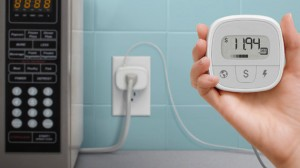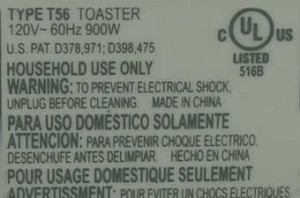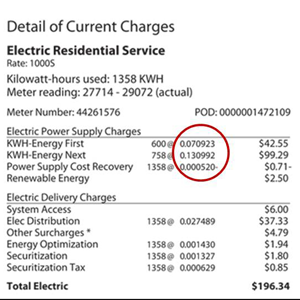By understanding how much money you are currently spending on your appliances and home electronics, you can find ways to cut costs and save money. Using the information found in this article, you will be able to calculate how much energy an appliance is using and, in turn, find ways to cut costs and make your home more energy efficient.

Estimating exactly how much electricity your appliance and home electronics use can be done in a few different ways:

• If the appliance or electronic has an Energy Guide label (not all do), you can review it for an estimate of the average energy consumption and cost of operation for that specific model.
• Electricity usage monitors will give you readings as to how much electricity an appliance or electronic is using.
• Use the formulas provided below to calculate the annual energy consumption and costs.
• Have a whole-house energy monitoring system installed.### How to Calculate Annual Electricity Consumption and Costs

To find the annual energy consumption and cost of an appliance or home electronic, use the steps below.

• First, get a general estimate of how many hours per day the appliance runs. There are two different ways to do this:
• Rough Estimate – If you know that you leave your living room lights on for 5 hours per day, then that is the number you will use. For an appliance like your refrigerator, which is turned “on” all of the time, but actually cycles on and off to maintain its interior temperature, take the total time the refrigerator is plugged in and divide by three. This will give you the number of hours it actually operates at its maximum wattage.
• Keep a Usage Log – For some appliances, such as an oven, microwave, computer, television, or dishwasher, you may want to keep a usage log. This will help you get accurate usage times.• Next, you must find the wattage of the appliance. Where can I find that, you may ask?
• Stamped on the appliance or electronic – Usually, most appliances and home electronics have the wattage stamped on the bottom or back, or have a nameplate with the information. The wattage shown is the maximum power used by the appliance. It is not uncommon for appliances to have a range of settings, meaning the actual power an appliance consumes will depend on the setting that is being utilized. For example, a hair dryer set to low will use less power than a hair dryer set on high.
• Use your Math skills – Sometimes, you may not be able to find the wattage listed on the appliance, but this does not mean that you are defeated! The way to do the estimate in this scenario is by finding the electrical current draw (listed in amperes) and multiplying it by the voltage that the appliance uses. In the United States, most appliances use 120 volts. However, larger appliances such as electric clothes dryers and electric stoves typically use 240 volts. You may find the amperes stamped on your appliance in place of the wattage. Another place to check is in the owner’s manual of the manufacturer’s website.
• Now, find the daily energy consumption by using the formula below:

(Wattage x Hours Used Per Day) ÷ 1000 = Daily Kilowatt-hour (kWh) consumption

• Then, find the annual energy consumption by using the formula below:

Daily kWh consumption x number of days used per year = annual energy consumption

• Finally, find the annual cost to run the appliance by using the formula below:

Annual energy consumption x utility rate per kWh = annual cost to run applianceExample: Now, let’s find the annual cost of operating a toaster by using the steps provided above.

1. Estimate of time used: The toaster is in use for approximately 15 minutes per day for breakfast, or 0.25.
2. Wattage: The wattage shown on the label is 900W.
3. Daily energy consumption:

900W x .25 ÷ 1000 = 0.225 kWh

1. Annual energy consumption: The toaster is used 7 days per week, 52 weeks per year, or 365 days per year.

0.225 kWh x 365 = 82.125 kWh

1. Annual cost to operate: The utility rate is 13 cents per kWh (see the image below to learn where to look for the utility rate on your bill).

82.125 kWh x \$0.13/kWh = \$10.68 per yearNow that you know how to calculate your appliance and home electronics’ energy use and cost per year, you can find out which appliances are costing you the most money and explore ways to save. If your appliances are not already Energy Star rated, investing in appliances that are is a good place to start. Energy Star was created in 1992 by the Environmental Protection Agency (EPA) and the Department of Energy. Products carrying the Energy Star label usually use 20-30% less energy than that required by federal standards. Another option is to set lights, fans, and other appliances on a timer in order to control the amount of electricity used when not around.